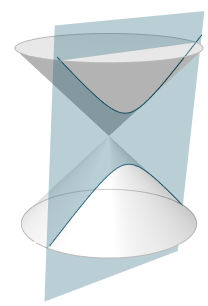Search IntMath
Close

450+ Math Lessons written by Math Professors and Teachers

5 Million+ Students Helped Each Year

1200+ Articles Written by Math Educators and Enthusiasts

Simplifying and Teaching Math for Over 23 Years

# Conic Sections - interactive 3-D graph

In the following interactive, you can vary parameters to produce the conics we learned about in this chapter.

In the applet, you'll see two cones joined at their apexes. Imagine these cones are of infinite height (but shown with a particular height here for practical reasons) so we can see the extended conic sections.

## Things to do

As you change sliders, observe the resulting conic type (either circle, ellipse, parabola, hyperbola or degenerate ellipse, parabola or hyperbola when the plane is at critical positions).

1. Change the θ-value, which changes the angle of the intersecting plane. (The angle is shown in radians and degrees.)
2. Change the y-value, which moves the intersecting plane left or right. (This will make more sense when the angle θ is not zero.)
3. Change the z-value, which moves the plane up or down.
4. Move around the 3D graph by dragging it up, down, left or right.
5. Zoom in and out using the mouse scroll wheel, or normal pinching on a mobile device
6. Pan the whole graph left, right, up or down using the right mouse button and dragging

Conic section: Ellipse

z
y

If for any reason you can't see the interactive applet above, here's a screen shot:Screen shot - conic-sections graph interactive

## Next up...

The next page describes the Polar Coordinate system:

The Polar Co-ordinate System »

## Problem SolverThis tool combines the power of mathematical computation engine that excels at solving mathematical formulas with the power of GPT large language models to parse and generate natural language. This creates math problem solver thats more accurate than ChatGPT, more flexible than a calculator, and faster answers than a human tutor. Learn More.